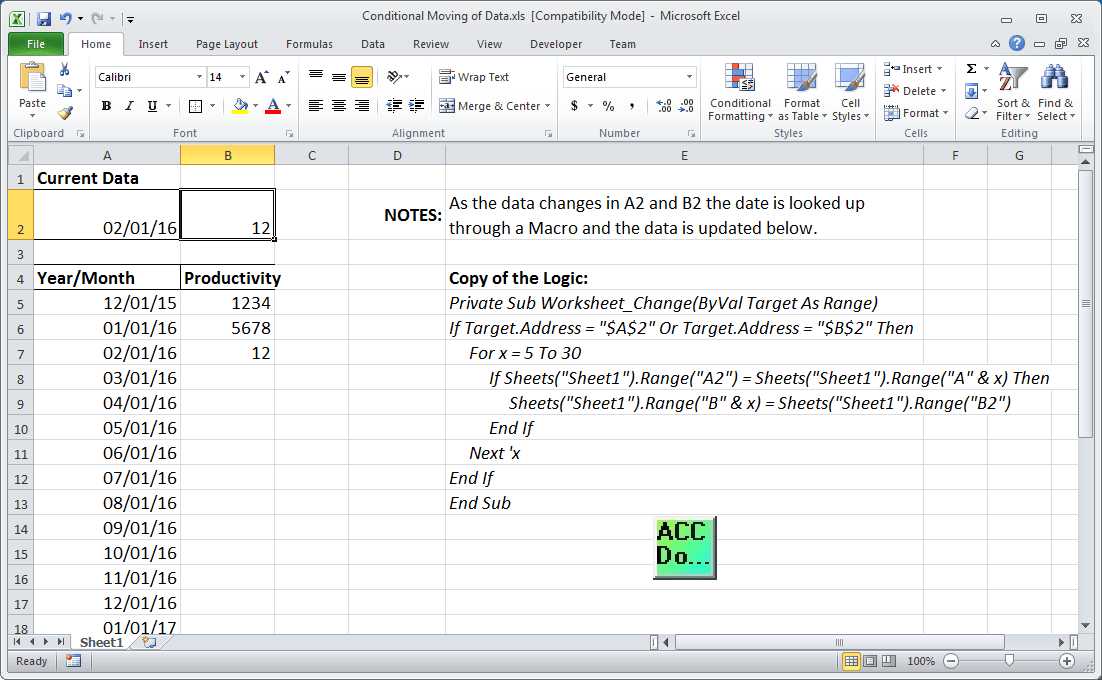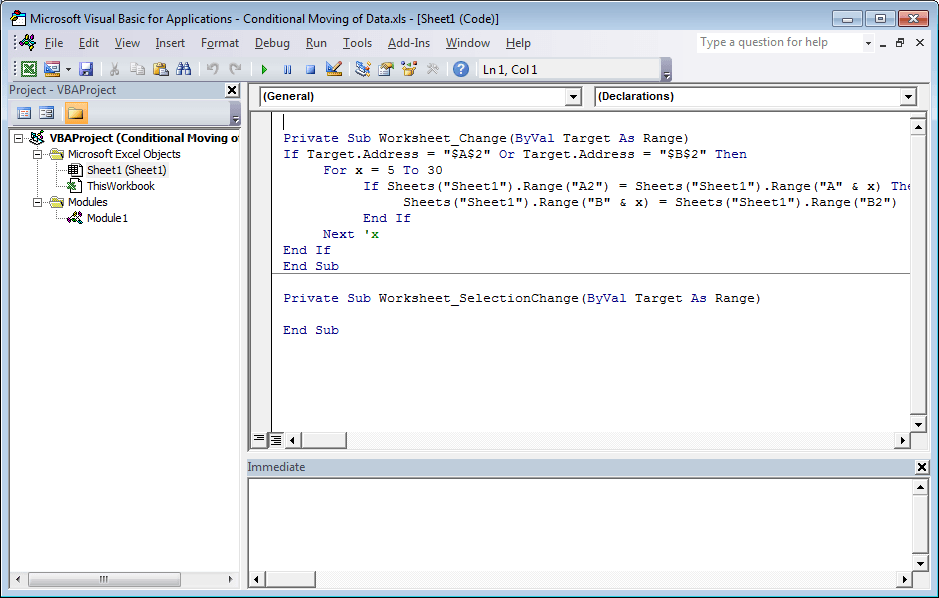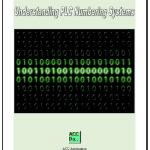Excel Conditional Movement of Data

I have been recently asked how you can move data conditionally on an excel spreadsheet once the information has been obtained from the PLC? Monthly data was being collected from the PLC and put on an excel spreadsheet using Omron CX-Server DDE. They wanted at the beginning of each month to move the data to the appropriate month so they can track the changes.

The following three blogs were posted on how to get data into the Microsoft Excel Spreadsheet from the programmable logic controller. (PLC)

HOW TO IMPLEMENT MODBUS TCP PROTOCOL USING VBA WITH EXCEL

IMPLEMENTING THE OMRON CX SERVER DDE AND EXCEL

HOW TO IMPLEMENT THE OMRON HOST LINK PROTOCOL PART 2 – VBA

The method to move the data around the spreadsheet once the data has been obtained will be done by Visual Basic for Applications. (VBA)

Make a spreadsheet in excel as follows:Operation:
When the current data in A2 or B2 changes, it will trigger a macro to execute. This macro will use the Worksheet_Change to trigger the code. The date in A2 is then compared to all of the dates located from A5 to A30. If a match is found then the value in B2 is placed beside the matching date found the column. Example: A2 = A7 then B7 = B2
Here is a look at the VBA editor and code:Here is the following code:
Private Sub Worksheet_Change(ByVal Target As Range)
For x = 5 To 30
If Sheets(“Sheet1”).Range(“A2”) = Sheets(“Sheet1”).Range(“A” & x) Then
Sheets(“Sheet1”).Range(“B” & x) = Sheets(“Sheet1”).Range(“B2”)
End If
Next ‘x
End If
End Sub

Watch on YouTube: Excel Conditional Movement of Data
Thank you,
Garry

If you’re like most of my readers, you’re committed to learning about technology. Numbering systems used in PLC’s are not difficult to learn and understand. We will walk through the numbering systems used in PLCs. This includes Bits, Decimal, Hexadecimal, ASCII and Floating Point.

To get this free article, subscribe to my free email newsletter.

Use the information to inform other people how numbering systems work. Sign up now.